×#### Thank you for registering.

One of our academic counsellors will contact you within 1 working day.

Click to Chat

1800-1023-196

+91-120-4616500

CART 0

• 0

MY CART (5)

Use Coupon: CART20 and get 20% off on all online Study Material

ITEM
DETAILS
MRP
DISCOUNT
FINAL PRICE
Total Price: Rs.

There are no items in this cart.
Continue Shopping• Complete JEE Main/Advanced Course and Test Series
• OFFERED PRICE: Rs. 15,900
• View Details

Practical Geometry Exercise 18.4

Question: 1

Construct a quadrilateral ABCD, in which AB = 6 cm, BC = 4 cm, CD = 4 cm, ∠B = 95° and ∠C = 90°.

Solution: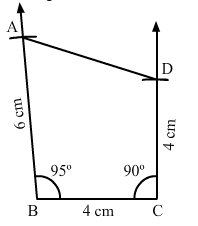Steps of construction:

Step I: Draw BC = 4 cm.

Step II: Construct ∠ABC = 95° at B.

Step III: With B as the center and radius 6 cm, cut off BA = 6 cm.

Step IV: Construct ∠BCD = 90° at C.

Step V: With C as the center and radius 4 cm, cut off BA = 4 cm.

Step VI: Join CD.

Question: 2

Construct a quadrilateral ABCD, where AB = 4.2 cm, BC = 3.6 cm, CD = 4.8 cm, ∠B = 30° and ∠C = 150°.

Solution:Steps of construction:

Step I: Draw BC = 3.6 cm.

Step II: Construct ∠ABC=30° at B.

Step III: With B as the center and radius 4.2 cm, cut off BA = 4.2 cm.

Step IV: Construct ∠BCD=150° at C.

Step V: With C as the center and radius 4.8 cm, cut off CD = 4.8 cm.

Question: 3

Construct a quadrilateral PQRS, in which PQ = 3.5 cm, QR = 2.5 cm, RS = 4.1 cm, ∠Q = 75° and ∠R = 120°.

Solution: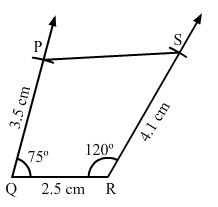Steps of construction:

Step I: Draw QR = 2.5 cm.

Step II: Construct ∠PQr=75° at Q.

Step III: With Q as the center and radius 3.5 cm, cut off QP = 3.5 cm.

Step IV: Construct ∠QRS=120° at R.

Step V: With R as the center and radius 4.1 cm, cut off RS = 4.1 cm.

Step VI: Join PS.

Question: 4

Construct a quadrilateral ABCD given BC = 6.6 cm, CD = 4.4 cm, AD = 5.6 cm and ∠D = 100° and ∠C = 95°.

Solution: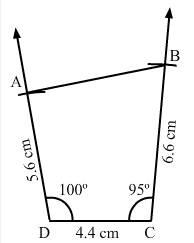Steps of construction:

Step I: Draw DC = 4.4 cm.

Step II: Construct ∠ADC=100° at D.

Step III: With D as the center and radius 5.6 cm, cut off DA = 5.6 cm.

Step IV: Construct ∠BCD=95° at C.

Step V: With C as the center and radius 6.6 cm, cut off CB = 6.6 cm.

Step VI: Join AB.

Question: 5

Construct a quadrilateral ABCD, in which AD = 3.5 cm, AB = 4.4 cm, BC = 4.7 cm, ∠A = 125° and ∠B = 120°.

Solution: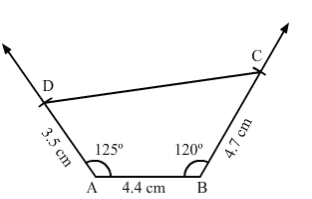Steps of construction:

Step I: Draw AB = 4.4 cm.

Step II: Construct ∠BAD = 125° at A.

Step III: With A as the centre and radius 3.5 cm, cut off AD = 3.5 cm.

Step IV: Construct ∠ABC = 125° at B.

Step V: With B as the centre and radius 4.7 cm, cut off BC = 4.7 cm.

Step VI: Join CD.

Question: 6

Construct a quadrilateral PQRS, in which ∠Q = 45°, ∠R = 70°, QR = 5 cm, PQ = 9 cm and RS = 7 cm.

Solution: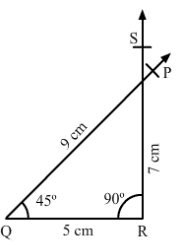Steps of construction:

Step I: Draw QR = 5 cm.

Step II: Construct ∠PQR=45° at Q.

Step III: With Q as the center and radius 9 cm, cut off QP = 9 cm.

Step IV: Construct ∠QRS=90° at R.

Step V: With R as the center and radius 7 cm, cut off RS = 7 cm.

Since, the line segment PQ and RS intersect each other, the quadrilateral cannot be constructed.

Question: 7

Construct a quadrilateral ABCD in which AB = BC = 3 cm, AD = 5 cm, ∠A = 90° and ∠B = 105°.

Solution: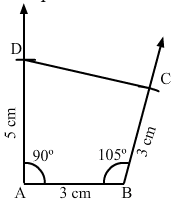Steps of construction:

Step I: Draw AB = 3 cm.

Step II: Construct ∠DAB=90° at A.

Step III: With A as the center and radius 5 cm, cut off AD = 5 cm.

Step IV: Construct ∠ABC=105° at B.

Step V: With B as the center and radius 3 cm, cut off BC = 3 cm.

Step VI: Join CD.

Question: 8

Construct a quadrilateral BDEF, where DE = 4.5 cm, EF = 3.5 cm, FB = 6.5 cm, ∠F = 50° and ∠E = 100°.

Solution:Steps of construction:

Step I: Draw EF = 3.5 cm.

Step II: Construct ∠DEF = 100° at E.

Step III: With E as the center and radius 4.5 cm, cut off DE = 4.5 cm.

Step IV: Construct ∠EFB = 50° at F.

Step V: With F as the center and radius 6.5 cm, cut off FB = 6.5 cm.

Step VI: Join BD.### Course Features

• 728 Video Lectures
• Revision Notes
• Previous Year Papers
• Mind Map
• Study Planner
• NCERT Solutions
• Discussion Forum
• Test paper with Video Solution